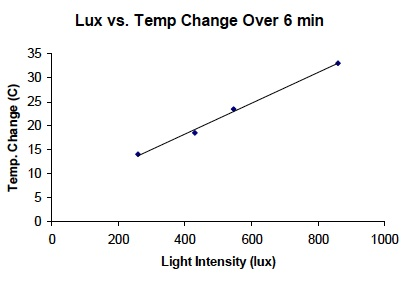# Investigation 2 – PostLab

### ANALYZE ITComplete the Analysis Questions in your SDR then discuss them as a class.

• What is the temperature change for each light bulb? To find the change in temperature subtract the starting temperature from the temperature at 6 minutes. Write your answers in the last column of Table C.

• What form of energy was light converted into in this experiment? Explain your answer.
• The temperature of a substance increases when particles of the substance move more quickly. The particles have more thermal energy. Is thermal energy (heat) a type of kinetic or potential energy? Why?
• Did different light bulbs result in different amounts of thermal energy (heat)? Is there a relationship between the wattage of light bulbs and thermal energy? Support the answer with data from the experiment.• Look at the sample graph above. How does the amount of light energy of each light bulb relate to the amount of heat energy in the air around each light bulb? Use data to support the answer.
• A straight line on the graph demonstrates a direct relationship between light energy and heat energy.
• This direct relationship indicates that energy was conserved in this system as it was transferred from light energy to heat energy.
• If energy was not conserved, the line would not be straight.

Note: Questions marked with a triangle (∆) are included to enrich your understanding. These questions do not appear in your SDR but should be used as additional discussion points during the PostLab.

Review the forms and types of energy observed in Investigation Two by considering the following questions:

• ∆ What forms of energy were used in this Investigation?
• ∆ Were these forms of energy kinetic energy, potential energy or both?
• ∆ Was energy converted in this experiment?

### GET FOCUSED

Complete the Focus Questions in your SDR then discuss them as a class.

• Can one form of energy be converted to another? Support your answer with data from your experiments.
• How does converting energy from one form to another relate to the conservation of energy?